SYLLABUS  Previous: 3.3 Improved model using  Up: 3.3 Improved model using  Next: 3.3.2 Itô lemma

3.3.1 Wiener process and martingales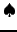[ SLIDE SDE - Wiener - Markov || VIDEO modem - LAN - DSL]

Although it is not possible to predict with any certainty the spot price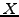of an asset in an efficient market, sect.2.1.1 demonstrated in that possible realizations can be simulated with their probability of occurrence by summing price increments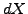over small steps in time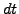. Separating the deterministic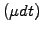from the remaining random component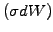, the evolution of the random variablesatisfies a stochastic differential equation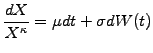(3.3.1#eq.1)

where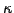=0 (alt. 1) chooses between the normal (alt. log-normal) distribution of increments discussed previously in (2.1.1#eq.1). Integrating over time, the first term yields a uniform (alt. exponential) growth with a rate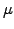that accounts for example for a continuous payment of a fixed dividend (alt. a compounded interest rate). The second term reproduces a random walk proportional to the market volatility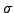, using a so-called Wiener process that has the following properties
1. The Wiener increment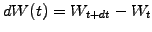over a time stepis a random variable drawn from a normal distribution with zero mean and a time-step variance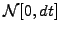(1.4#eq.5). Extensions to other distributions (e.g. 2.1.1#eq.3) are possible.
2. The Wiener increment is independent of the past. Using the definition (1.4#eq.3) for the correlation, this is satisfied when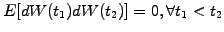.
A convenient way of writing this is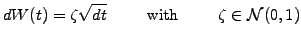(3.3.1#eq.2)

where different realizations of the random variable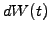are generated using random numbers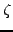that are normally distributed. This construction provides the mathematical foundation for the Monte-Carlo simulations, where the mean value of the increment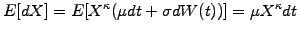(3.3.1#eq.3)

and the variance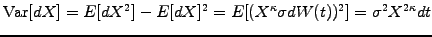(3.3.1#eq.4)

are matched with historical data to forecast possible evolutions into the future. Third or even higher order moments of the probability distribution can in principle also be matched; experience, however, shows that little is to be gained from such a procedure. A better description of the market is obtained by performing a principal components analysis , where several imperfectly correlated random variables are identified and then superposed to drive increments of the form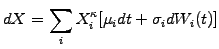(3.3.1#eq.5)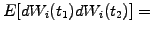0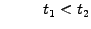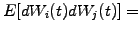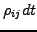(3.3.1#eq.6)

where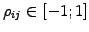is a correlation factor. The first component typically accounts for 80-90% (and the first three for up to 95-99%) of the variance observed in the market price of forward rates .
Note that the forecast value for the price of a share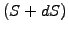or an interest rate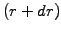constructed using Wiener increments depends only the present value: this independence from the past is known in mathematics as the Markov property. Also note that a suitable choice of a numeraire can always be found to normalize random variables and make them risk-neutral by scaling out the drift observed in the real world: called martingales, such variables play an important role in the construction of financial models.

SYLLABUS  Previous: 3.3 Improved model using  Up: 3.3 Improved model using  Next: 3.3.2 Itô lemma